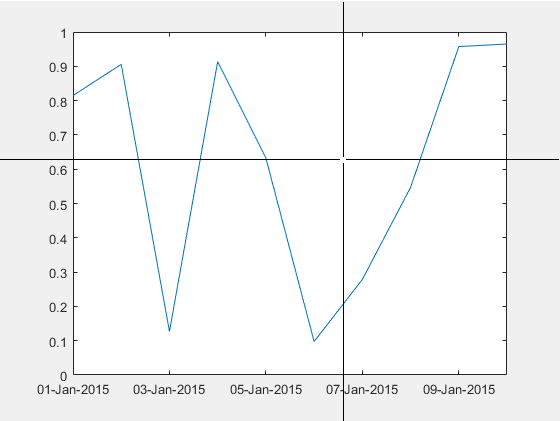Documentation

# num2ruler

Convert numeric data for use with specific ruler

## Syntax

``data = num2ruler(num,ruler)``

## Description

example

````data = num2ruler(num,ruler)` converts the numeric values in `num` to the appropriate values for the specified ruler. The resulting values depend on both the type and limits of the `ruler` input. The output `data` is an array the same size as `num`.```

## Examples

collapse all

Convert the output of `ginput` from a numeric value to the equivalent date value.

Create a plot with dates along the x-axis. Then, click a point in the axes and use the `ginput` function to return the location of the selected point.

```t = datetime(2015,1,1:10); y = rand(10,1); plot(t,y) ax = gca; [x,y] = ginput(1)``````x = 5.8168 y = 0.6458```

The `ginput` function returns the location as numeric values. Use `num2ruler` to determine the date value that is equivalent to the numeric value returned for `x`.

`xdate = num2ruler(x,ax.XAxis)`
```xdate = 06-Jan-2015 19:36:13```

## Input Arguments

collapse all

Numeric data, specified as an array.

Example: `num = [1 2 3]`

Ruler that determines data mapping, specified as one of these types of ruler objects:

• `DurationRuler` object — Convert the input `num` to duration values.

• `DatetimeRuler` object — Convert the input `num` to datetime values.

• `NumericRuler` object — No conversion. For this case, the output `data` is equal to the input `num`.

• `GeographicRuler` object — No conversion. For this case, the output `data` is equal to the input `num`.

Specify the ruler object by referring to either the `XAxis`, `YAxis`, or `ZAxis` property of the `Axes` object.

```ax = gca; data = num2ruler(num,ax.XAxis)```